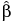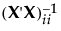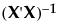Estimation Efficiency Outline
 • The length of the ideal confidence interval for the parameter is subtracted from the length of its actual confidence interval.
 • This difference is then divided by the length of the ideal confidence interval.
The covariance matrix for the ordinary least squares estimator is σ2(XX)-1. The diagonal elements of (XX)-1 are the relative variances (the variances divided by σ2) of the parameter estimates. For two-level designs and using the effects coding convention (see Coding in Column Properties), the minimum value of the relative variance for any parameter estimate is 1/n, where n is the number of runs. This occurs when all the effects for the design are orthogonal and the design is D-optimal.
Letdenote the vector of parameter estimates. The ideal design, which may not exist, is a design whose covariance matrix is given as follows:
whereis the n by n identity matrix and σ is the standard deviation of the response.
Specifically, for the ith parameter estimate, the Fractional Increase in Confidence Interval Length is defined as follows:
σ2 is the unknown response variance,
X is the model matrix for the given design, defined in The Alias Matrix in Technical Details,is the ith diagonal entry of, and
n is the number of runs.
X is the model matrix defined in The Alias Matrix in Technical Details, andis the ith diagonal entry of.

Help created on 9/19/2017